Next: Vector Triple Product Up: Vector Algebra and Vector Previous: Rotation

Scalar Triple Product

Consider three vectors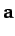,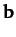, and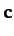. The scalar triple product is defined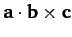. Now,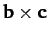is the vector area of the parallelogram defined byand. So,is the scalar area of this parallelogram multiplied by the component ofin the direction of its normal. It follows thatis the volume of the parallelepiped defined by vectors,, and--see Figure A.106. This volume is independent of how the triple product is formed from,, and, except that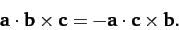(1310)

So, the volume'' is positive if,, andform a right-handed set (i.e., iflies above the plane ofand, in the sense determined from a right-hand circulation rule by rotatingonto) and negative if they form a left-handed set. The triple product is unchanged if the dot and cross product operators are interchanged,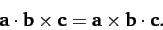(1311)

The triple product is also invariant under any cyclic permutation of,, and,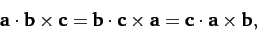(1312)

but any anti-cyclic permutation causes it to change sign,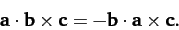(1313)

The scalar triple product is zero if any two of,, andare parallel, or if,, andare coplanar.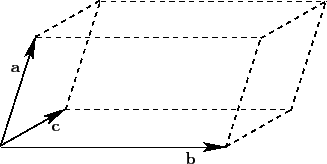If,, andare non-coplanar then any vector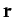can be written in terms of them: i.e.,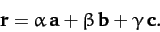(1314)

Forming the dot product of this equation with, we then obtain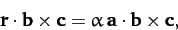(1315)

so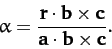(1316)

Analogous expressions can be written for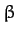and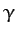. The parameters,, andare uniquely determined provided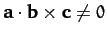: i.e., provided that the three vectors are non-coplanar.Next: Vector Triple Product Up: Vector Algebra and Vector Previous: Rotation
Richard Fitzpatrick 2011-03-31